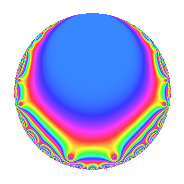# Properties

 Label 2004.1.g.cLevel 2004 Weight 1 Character orbit 2004.g Self dual Yes Analytic conductor 1.000 Analytic rank 0 Dimension 2 Projective image $$D_{4}$$ CM disc. -2004 Inner twists 4

# Related objects

## Newspace parameters

 Level: $$N$$ = $$2004 = 2^{2} \cdot 3 \cdot 167$$ Weight: $$k$$ = $$1$$ Character orbit: $$[\chi]$$ = 2004.g (of order $$2$$ and degree $$1$$)

## Newform invariants

 Self dual: Yes Analytic conductor: $$1.00012628532$$ Analytic rank: $$0$$ Dimension: $$2$$ Coefficient field: $$\Q(\sqrt{2})$$ Coefficient ring: $$\Z[a_1, \ldots, a_{5}]$$ Coefficient ring index: $$1$$ Projective image $$D_{4}$$ Projective field Galois closure of 4.2.24048.2 Artin image size $$16$$ Artin image $D_8$ Artin field Galois closure of 8.2.96577152768.2

## $q$-expansion

Coefficients of the $$q$$-expansion are expressed in terms of $$\beta = \sqrt{2}$$. We also show the integral $$q$$-expansion of the trace form.

 $$f(q)$$ $$=$$ $$q$$ $$- q^{2}$$ $$+ q^{3}$$ $$+ q^{4}$$ $$-\beta q^{5}$$ $$- q^{6}$$ $$- q^{8}$$ $$+ q^{9}$$ $$+O(q^{10})$$ $$q$$ $$- q^{2}$$ $$+ q^{3}$$ $$+ q^{4}$$ $$-\beta q^{5}$$ $$- q^{6}$$ $$- q^{8}$$ $$+ q^{9}$$ $$+ \beta q^{10}$$ $$+ q^{12}$$ $$-\beta q^{15}$$ $$+ q^{16}$$ $$+ \beta q^{17}$$ $$- q^{18}$$ $$-\beta q^{20}$$ $$- q^{24}$$ $$+ q^{25}$$ $$+ q^{27}$$ $$+ \beta q^{30}$$ $$- q^{32}$$ $$-\beta q^{34}$$ $$+ q^{36}$$ $$+ \beta q^{40}$$ $$+ \beta q^{41}$$ $$-\beta q^{43}$$ $$-\beta q^{45}$$ $$+ q^{48}$$ $$+ q^{49}$$ $$- q^{50}$$ $$+ \beta q^{51}$$ $$+ \beta q^{53}$$ $$- q^{54}$$ $$-\beta q^{60}$$ $$+ q^{64}$$ $$+ \beta q^{67}$$ $$+ \beta q^{68}$$ $$- q^{72}$$ $$+ q^{75}$$ $$+ \beta q^{79}$$ $$-\beta q^{80}$$ $$+ q^{81}$$ $$-\beta q^{82}$$ $$-2 q^{85}$$ $$+ \beta q^{86}$$ $$+ \beta q^{90}$$ $$- q^{96}$$ $$-2 q^{97}$$ $$- q^{98}$$ $$+O(q^{100})$$ $$\operatorname{Tr}(f)(q)$$ $$=$$ $$2q$$ $$\mathstrut -\mathstrut 2q^{2}$$ $$\mathstrut +\mathstrut 2q^{3}$$ $$\mathstrut +\mathstrut 2q^{4}$$ $$\mathstrut -\mathstrut 2q^{6}$$ $$\mathstrut -\mathstrut 2q^{8}$$ $$\mathstrut +\mathstrut 2q^{9}$$ $$\mathstrut +\mathstrut O(q^{10})$$ $$2q$$ $$\mathstrut -\mathstrut 2q^{2}$$ $$\mathstrut +\mathstrut 2q^{3}$$ $$\mathstrut +\mathstrut 2q^{4}$$ $$\mathstrut -\mathstrut 2q^{6}$$ $$\mathstrut -\mathstrut 2q^{8}$$ $$\mathstrut +\mathstrut 2q^{9}$$ $$\mathstrut +\mathstrut 2q^{12}$$ $$\mathstrut +\mathstrut 2q^{16}$$ $$\mathstrut -\mathstrut 2q^{18}$$ $$\mathstrut -\mathstrut 2q^{24}$$ $$\mathstrut +\mathstrut 2q^{25}$$ $$\mathstrut +\mathstrut 2q^{27}$$ $$\mathstrut -\mathstrut 2q^{32}$$ $$\mathstrut +\mathstrut 2q^{36}$$ $$\mathstrut +\mathstrut 2q^{48}$$ $$\mathstrut +\mathstrut 2q^{49}$$ $$\mathstrut -\mathstrut 2q^{50}$$ $$\mathstrut -\mathstrut 2q^{54}$$ $$\mathstrut +\mathstrut 2q^{64}$$ $$\mathstrut -\mathstrut 2q^{72}$$ $$\mathstrut +\mathstrut 2q^{75}$$ $$\mathstrut +\mathstrut 2q^{81}$$ $$\mathstrut -\mathstrut 4q^{85}$$ $$\mathstrut -\mathstrut 2q^{96}$$ $$\mathstrut -\mathstrut 4q^{97}$$ $$\mathstrut -\mathstrut 2q^{98}$$ $$\mathstrut +\mathstrut O(q^{100})$$

## Character Values

We give the values of $$\chi$$ on generators for $$\left(\mathbb{Z}/2004\mathbb{Z}\right)^\times$$.

 $$n$$ $$673$$ $$1003$$ $$1337$$ $$\chi(n)$$ $$-1$$ $$-1$$ $$-1$$

## Embeddings

For each embedding $$\iota_m$$ of the coefficient field, the values $$\iota_m(a_n)$$ are shown below.

For more information on an embedded modular form you can click on its label.

Label $$\iota_m(\nu)$$ $$a_{2}$$ $$a_{3}$$ $$a_{4}$$ $$a_{5}$$ $$a_{6}$$ $$a_{7}$$ $$a_{8}$$ $$a_{9}$$ $$a_{10}$$
2003.1
 1.41421 −1.41421
−1.00000 1.00000 1.00000 −1.41421 −1.00000 0 −1.00000 1.00000 1.41421
2003.2 −1.00000 1.00000 1.00000 1.41421 −1.00000 0 −1.00000 1.00000 −1.41421
 $$n$$: e.g. 2-40 or 990-1000 Significant digits: Format: Complex embeddings Normalized embeddings Satake parameters Satake angles

## Inner twists

Char. orbit Parity Mult. Self Twist Proved
1.a Even 1 trivial yes
2004.g Odd 1 CM by $$\Q(\sqrt{-501})$$ yes
12.b Even 1 yes
167.b Odd 1 yes

## Hecke kernels

This newform can be constructed as the intersection of the kernels of the following linear operators acting on $$S_{1}^{\mathrm{new}}(2004, [\chi])$$:

 $$T_{5}^{2}$$ $$\mathstrut -\mathstrut 2$$ $$T_{7}$$ $$T_{11}$$ $$T_{179}$$ $$\mathstrut -\mathstrut 2$$